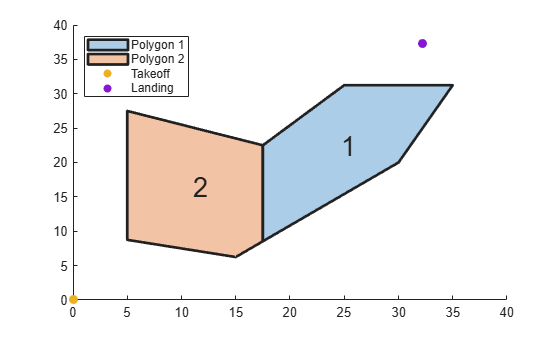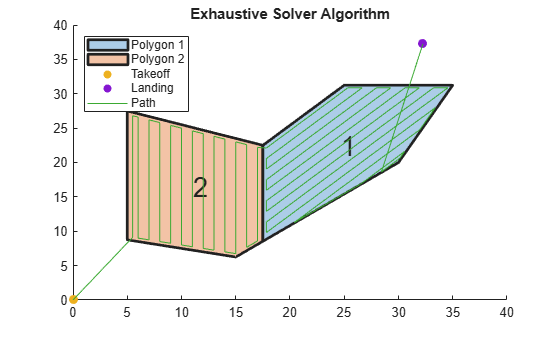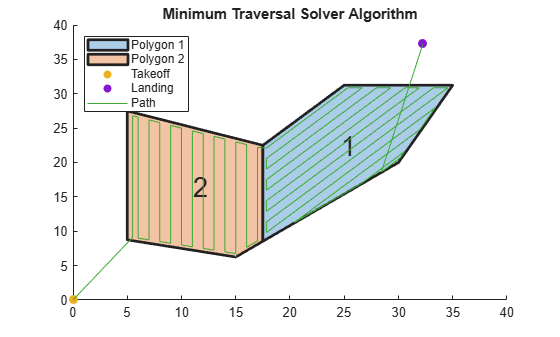# coverageDecomposition

Decompose concave polygon into convex polygons

Since R2023a

## Syntax

``polygons = coverageDecomposition(concavePolygon)``
``polygons = coverageDecomposition(concavePolygon,IntersectionTol=tolerance)``

## Description

The `coverageDecomposition` function decomposes coverage regions into subregions using vertex-edge decomposition. This enables you to plan more efficient coverage paths using optimized planning options for each subregion to have minimum turns and then connect the plans together.

example

````polygons = coverageDecomposition(concavePolygon)` decomposes the concave polygon `concavePolygon` into convex polygon areas `polygons` based on minimum-width criteria.```
````polygons = coverageDecomposition(concavePolygon,IntersectionTol=tolerance)` tests the vertex edge decompositions with slopes that are between the tolerance `tolerance`, which respect to the polygon edges.```

## Examples

collapse all

This example shows how to plan a coverage path for a region in local coordinates and compares the results of using the exhaustive solver with the results of using the minimum traversal solver.

Define the vertices for a coverage space.

`area = [5 8.75; 5 27.5; 17.5 22.5; 25 31.25; 35 31.25; 30 20; 15 6.25];`

Because vertices define a concave polygon and the coverage planner requires convex polygons, decompose the polygon into convex polygons. Then create a coverage space with the polygons from decomposition.

```polygons = coverageDecomposition(area); cs = uavCoverageSpace(Polygons=polygons);```

Define the takeoff and landing positions at `[0 0 0]` and `[32.25 37.25 0]`, respectively. Then show the coverage space and plot the takeoff and landing positions.

```takeoff = [0 0 0]; landing = [32.25 37.25 0]; show(cs); exampleHelperPlotTakeoffLandingLegend(takeoff,landing)```Create a coverage planner with the exhaustive solver algorithm and another coverage planner with a minimum traversal solver algorithm. Because `Polygon 2` is closer to the takeoff position, set the visiting sequence of the solver parameters such that we traverse `Polygon 2` first.

```cpeExh = uavCoveragePlanner(cs,Solver="Exhaustive"); cpMin = uavCoveragePlanner(cs,Solver="MinTraversal"); cpeExh.SolverParameters.VisitingSequence = [2 1]; cpMin.SolverParameters.VisitingSequence = [2 1];```

Plan with both solver algorithms using the same takeoff and landing positions.

```[wptsExh,solnExh] = plan(cpeExh,takeoff,landing); [wptsMin,solnMin] = plan(cpMin,takeoff,landing);```

Show the planned path for both the exhaustive and the minimum traversal algorithms.

```figure show(cs); title("Exhaustive Solver Algorithm") exampleHelperPlotTakeoffLandingLegend(takeoff,landing,wptsExh)``````figure show(cs); title("Minimum Traversal Solver Algorithm") exampleHelperPlotTakeoffLandingLegend(takeoff,landing,wptsMin)```Export the waypoints from the minimum traversal solver to a `.waypoints` file with the reference frame set to north-east-down.

`exportWaypointsPlan(cpMin,solnMin,"coveragepath.waypoints",ReferenceFrame="NED")`

## Input Arguments

collapse all

Concave polygon to decompose, specified as an N-by-2 matrix.

Example: `[0 0; 0 1; 1 1; 1 0]`

Data Types: `double`

Intersection tolerance, specified nonnegative numeric scalar. The `coverageDecomposition` function uses the intersection tolerance to test if the vertex-edge decompositions are valid. For example, when the `coverageDecomposition` function decomposes a polygon into two polygons, those two polygons now have edges that intersect each other. The slopes of these intersecting edges must be within this tolerance. This is useful when polygons are slightly off alignment.

Example: `[0 0; 0 1; 1 1; 1 0]`

Data Types: `single` | `double`

## Output Arguments

collapse all

Convex polygons, returned as a N-element cell array of M-by-2 matrices. N is the total number of polygons, and M is the total number of vertices that define each polygon.

The format of the vertices is local xy-coordinates in the form [x y], in meters.

Example: `{[0 0; 0 1; 1 1; 1 0],[1 1; 2 2; 3 1]}`

 Li, Yan, Hai Chen, Meng Joo Er, and Xinmin Wang. “Coverage Path Planning for UAVs Based on Enhanced Exact Cellular Decomposition Method.” Mechatronics 21, no. 5 (August 2011): 876–85. https://doi.org/10.1016/j.mechatronics.2010.10.009.